If you ever use LaTeX to write your research papers or technical reports, then you should know how useful and powerful this tool is. Let me just mention three well-known benefits of using LaTeX: 1) beautiful typeset output (especially when dealing with mathematical notations); 2) consistent handling of references and bibliography (thanks to BibTeX; 3) separation of content and style, meaning that all you need to write is plain text (also true for tables and figures, thanks to TikZ). LaTeX has a powerful markup language for document creation. One of the best and often misleading advantages of using this language is that it allows the creation of custom macros. These are user-defined commands that allows avoiding typing repetitive LaTeX commands (e.g., formatting strings or numbers) and even perform live computations (e.g., calculating numeric expression). Let me show you how it works!

# Creating a Simple User Custom Command

To add a custom command in your document, use \newcommand{\name}[num]{definition}. The following commands are examples that allow simplifying the use of some common English abbreviations:

Once added in your document, you just need to type \ie and the text i.e. will be printed in your document. Note that you need to use \@\xspace to indicate that a white space follows the displayed text.

# Inserting Author Notes

I often find very useful defining the \cesar and \todo commands in all my documents to create quick author notes and remarks in my documents. The following code defines these commands, which are very useful when inserting notes (e.g., \cesar{refine this sentence}):

For example, the \cesar{An author note} produces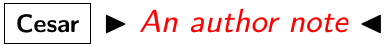, whereas \todo{A todo note} produces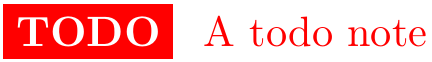.

# Formatting Numbers

You can use the numprint and fp packages to create your own commands to format numeric values. The following example creates a \np command to print numeric values in math mode, nicely formatted, and rounded by two decimal digits.

Now, for example, you can use \np{22.324} to print 22.32, or \np{22.324}{\%} to print 22.32%.

# Computing Numbers

You can create numeric variables using \def. This is very handy since you can keep all the values in your document in the same place. Furthermore, you can use \FPeval to compute values based on these variables. With \FPeval, your document will lively update every time you update some value. The following is a basic example:

In this example, using \nbSum prints the value 84 in the document.

# Handling Percentages

If you use percentages in your document, you can calculate a percentage directly based on two variables: a number and the total. The following \ShowPercentage command calculates rates based on two variables.

For example, \ShowPercentage{1221}{20023} prints 6.10%. Note that a percentage rounded to 0 will be presented as <1%

# Handling Ratios

Sometimes percentages are hard to read (e.g., when the numerator and denominator are large numbers). In such cases, you can present ratios instead. For example, to say that 4 is two times larger that 2. The following \Ratio command calculates based based on two variables.

For the previous example, it prints: 2x.

# Embedding Small Barcharts

A nice way to compare percentages in a graphical manner (especially in tables), is by embedding small barchart plots within them. The following \ChartSmall commands does the trick for you:

Note that you can use this command in three different flavors:

• \ChartSmall[x]{80}{155} produces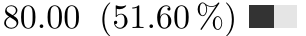• \ChartSmall[q]{80}{155} produces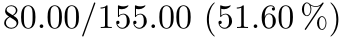• \ChartSmall[p]{80}{155} produces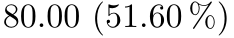# Executing Python Scripts in a LaTeX Document

You can leverage the computational capabilities of the Python programming language to compute values or automatize the creation of a LaTeX document. Here is an example, credited to this answer on StackExchange.

Here is file mypy.py called within the LaTeX document:

The output is as following: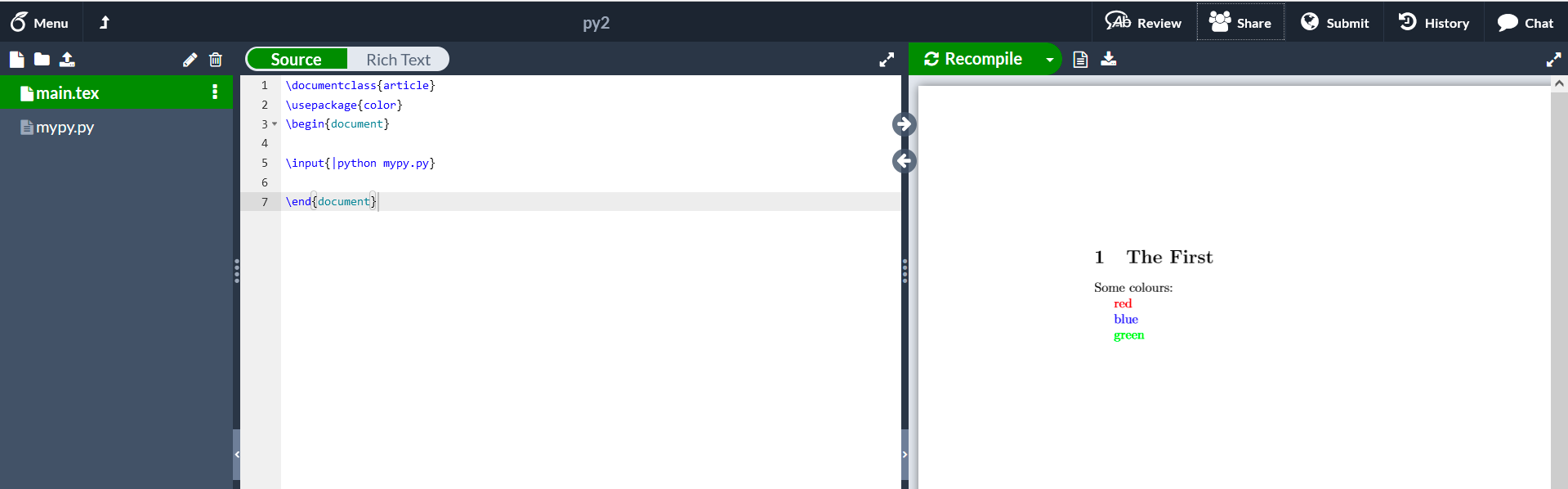# Conclusion

LaTeX is not only an awesome tool to create beautiful documents. It also provides packages to format and compute numeric values as variables. I’ve used all the custom commands presented in this post to ease the writing process of my research papers. Since then, dealing with numeric values and percentages has never been easier.